Definitions of Square Dance Calls and Concepts
Follow To A Diamond [C3A]Index -->  Plus  |  A1  |  A2  |  C1  |  C2  |  C3A  |  C3B  |  C4  |  NOL  |
Definitions (Text Only) -->  Plus  |  A1  |  A2  |  C1  |  C2  |  C3A  |  C3B  |  C4  |  NOL  |
 Find call:

 \$B8@8l(BMini Wave \$B\$N(B Box \$B\$^\$?\$OE,@Z\$J(B T-Bone \$B\$N(B 2 x 2 \$B\$+\$i!%(B

Trailers \$B\$O(B Follow Your Neighbor & Spread \$B\$r(B, Leaders \$B\$O(B Box Circulate once and a half \$B\$r\$7\$^\$9!%(B

\$B1&\$^\$?\$O:8\$N(B Mini Wave \$B\$N(B Box \$B\$+\$i\$O(B Diamond \$B\$G=*\$o\$j(B, T-Bone \$B\$N(B 2 x 2 \$B\$+\$i\$OIaDL(B Wave \$B\$G=*\$o\$j\$^\$9!%(B

Mini-Wave Box \$B\$+\$i(B: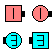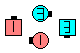Follow To A Diamond\$B\$NA0(B \$B8e(B

T-Bone 2 x 2 \$B\$+\$i(B: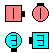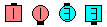Follow To A Diamond\$B\$NA0(B \$B8e(B

Follow To A(n) formation [C4]: \$BJ?9T\$J(B Waves \$B\$+\$i!%(B Trailers \$B\$O(B Follow Your Neighbor & Spread \$B\$r(B, Leaders \$B\$O(B 1/2 Split Circulate \$B\$r\$7\$F(B Phantom formation \$B\$G(B Circulate \$B\$r\$7\$^\$9!%(B Formation \$B\$O(B Hourglass, Interlocked Diamond, \$B\$^\$?\$O(B Magic Diamond \$B\$J\$I\$G\$9!%(B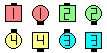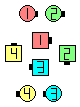Follow To An Hourglass\$B\$NA0(B \$B8e(B

Cross Follow To A Diamond [C4]: Mini Wave \$B\$N(B Box \$B\$^\$?\$OE,@Z\$J(B T-Bone \$B\$N(B 2 x 2 \$B\$+\$i!%(B Trailers \$B\$O(B Cross Your Neighbor & Spread \$B\$r(B, Leaders \$B\$O(B Box Circulate once and a half \$B\$r\$7\$^\$9!%(B Mini Wave \$B\$N(B Box \$B\$+\$i\$O(B Facing Diamond \$B\$G=*\$o\$j\$^\$9!%(B

Criss Cross Follow To A Diamond [C4]: Mini Wave \$B\$N(B Box \$B\$^\$?\$OE,@Z\$J(B T-Bone \$B\$N(B 2 x 2 \$B\$+\$i!%(B Trailers \$B\$O(B Cross Your Neighbor & Spread \$B\$r(B, Leaders \$B\$O(B Box Circulate \$B\$r\$7\$F(B Cross Extend \$B\$r\$7\$^\$9!%(B Mini Wave \$B\$N(B Box \$B\$+\$i\$O(B \$BH?BP\$NScatter Follow To A Diamond [C4]: \$BJ?9T\$J(B Waves (\$B\$^\$?\$OE,@Z\$J(B 2 x 4 \$B\$N(B T-Bone) \$B\$+\$i!%(B Trailers \$B\$O(B Follow Your Neighbor & Spread \$B\$r(B, Leaders \$B\$O(B (all 8) Circulate once and a half \$B\$r\$7\$^\$9!%(B \$BJ?9T\$J(B Waves \$B\$+\$i\$O(B Twin Diamonds \$B\$G=*\$o\$j\$^\$9!%(BCALLERLAB definition for Follow To A DiamondChoreography for Follow To A DiamondComments? Questions? Suggestions?